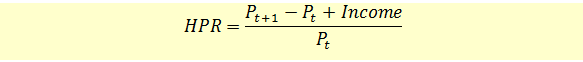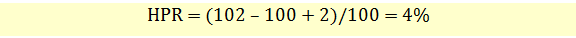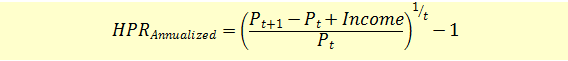# Holding Period Return (Total Return)

For investments, the Holding Period Return (HPR) refers to the total return earned from an investment or an investment portfolio over the holding period, that is, the period for which the asset or portfolio was held by the investor. The holding period can be anything such as 1 day, 1 month, 6 months, 1 year, 5 years and so on.

If you buy an asset now at $100 and sell it at$120 after 2 years, the holding period return will be (120 – 100)/100 = 20%. The time when the asset was bought can be labelled t and the current time when the asset is sold can be labelled t+1. If the asset pays an        y income such as dividend income on maturity, then that should also be added to the total returns. If P represents the price of the asset, then the holding period return formula can be presented as follows:Let’s take a simple example to understand the HPR calculation.

Let’s say that we purchased one share of a stock for $100 at the beginning of the year. After three months, the stock price has gone up to$102 and it also pays a dividend of \$2. The holding period return will be:The holding period returns can be annualized from either longer periods or shorter periods.

If the original HPR is calculated over multiple years, then the annualized returns can be calculated as follows: